# Linear Momentum, Angular Momentum and Kinetic Energy of Rigid Bodies (Part - 2) Civil Engineering (CE) Notes | EduRev

## Civil Engineering (CE) : Linear Momentum, Angular Momentum and Kinetic Energy of Rigid Bodies (Part - 2) Civil Engineering (CE) Notes | EduRev

The document Linear Momentum, Angular Momentum and Kinetic Energy of Rigid Bodies (Part - 2) Civil Engineering (CE) Notes | EduRev is a part of the Civil Engineering (CE) Course Introduction to Dynamics and Vibrations- Notes, Videos, MCQs.
All you need of Civil Engineering (CE) at this link: Civil Engineering (CE)

6.4.1 Deriving the linear momentum formula

By definition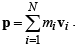We ca re-write this as follows: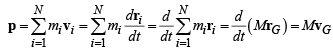(we used the definition of the COM to get the last result)

6.4.2 Deriving the angular momentum formula

Start with the definition: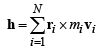Note that ri =rG + di and recall the relative velocity formula vi −vG = ω × (ri− rG) = ω ×di .  This means we can re-write the angular momentum as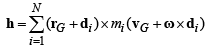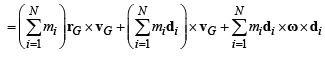Note that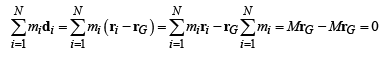Finally, recall the dreaded triple cross product formula

a × b × c= (a ⋅ c)b − (a ⋅b)c

This means that

di × ω× di = (di ⋅ di )ω − di (di ⋅ ω)

We can expand this out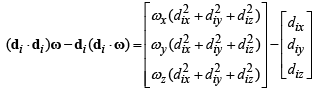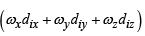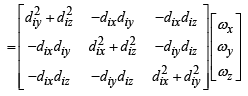This shows that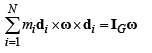Finally collecting terms gives the required answer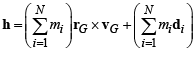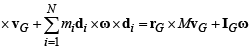6.4.3 Deriving the kinetic energy formula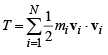We can use vi −vG = ω × (ri− rG) = ω ×di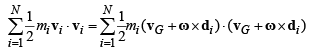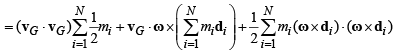Recall that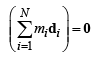and expand the dot product of two cross products using the formula

(a × b) ⋅ (c × d) = (a ⋅c)(b ⋅ d) − (b ⋅ c)(a ⋅d)

This shows that

(ω x di) • (ω x di) = (ω • ω)(di • di) - (w • di)2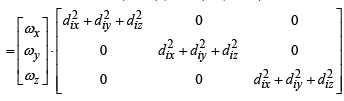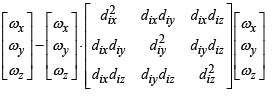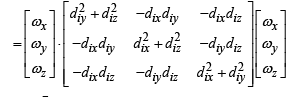= ω ⋅IG ω

6.4.5 Calculating the center of mass and inertia of a general rigid body

It is not hard to extend the results for a system of N particles to a general rigid body. We simply regard the body to be made up of an infinite number of vanishingly small particles, and take the limit of the sums as the particle volume goes to zero. The sums all turn into integrals.

3D problems:  For a body with mass density ρ (mass per unit volume) we have that

• The total mass is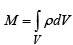• The position of the center of mass is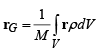• The mass moment of inertia about the center of mass is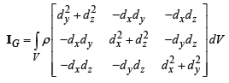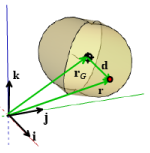where d = r − rG

For 2D problems: We know the COM must lie in the i,j plane and we don’t need to calculate the whole matrix.

For a body with mass per unit area µ we can therefore use the formulas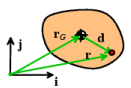• The total mass is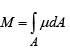• The position of the center of mass is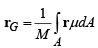• The mass moment of inertia about the center of mass is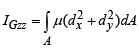where d = r − rG

Example 1: To show how to use these, let’s calculate the total mass, center of mass, and mass moment of inertia of a rectangular prism with faces perpendicular to the i, j,k axes: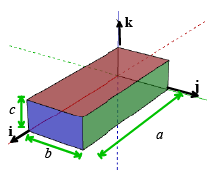First the total mass (sort of trivial)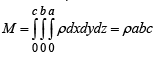Now the COM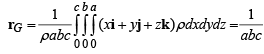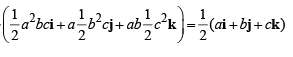And finally the mass moment of inertia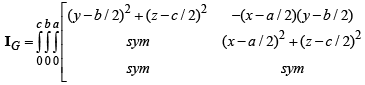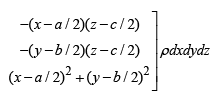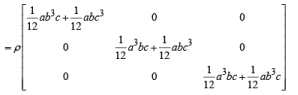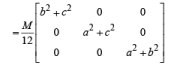Example 2: As a second example, let’s calculate the mass moment of inertia of a cylinder with mass density ρ , length L and radius a.  (We know the COM is at the center and we know the total mass so we won’t bother calculating those). We have to do the integral with polar coordinates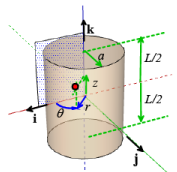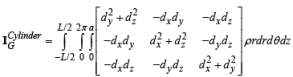Now note that d =r cosθ dy = r sinθ dz = z .  We can have Mupad do the dirty work:

[reset() :

[ds := r*cos(q): dy := r*sin(q): dz := z: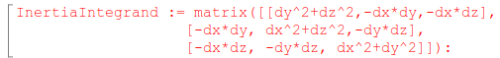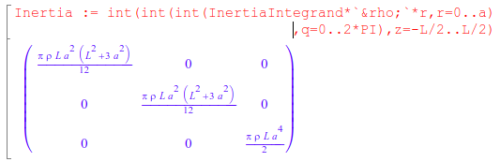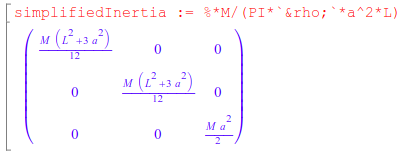Example 3: Let’s finish up with a 2D example. Find the mass, center of mass, and out of plane mass moment of inertia of the triangle shown in the figure.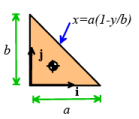The total mass is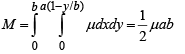The position of the COM is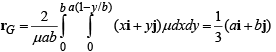The 2D mass moment of inertia is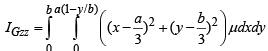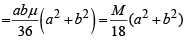This is all a big pain, and you may be contemplating a life of crime instead of an engineering career.   Fortunately, it is very rare to have to do these sorts of integrals in practice, because all the integrals for common shapes have already been done. You can google most of them. The tables below give a short list of all the objects we will encounter in this course.

Table of mass moment of inertia tensors for selected 3D objects

 PrismM = ρ abc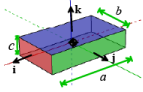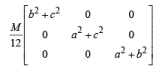Solid CylinderM = πρ a2L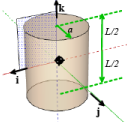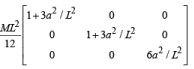Solid ConeM =π/h ρα2h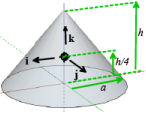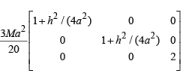Solid SphereM = 4/3 πρα3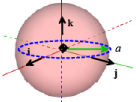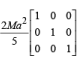Solid EllipsoidM =4/3 πραbc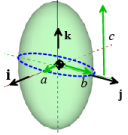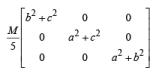Hollow CylinderM = πρ (b2 − a2)L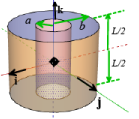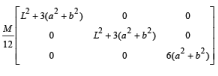Table of mass moment of inertia about perpendicular axis for selected 2D objects

 Square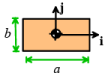IGzz = M/12 (α2 + b2) Disk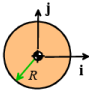IGzz = M/2R2 Thin ring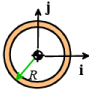IGzz = -MR2 Hollow disk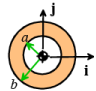IGzz = M/2 (α2 + b2) Slender rod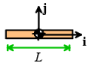IGzz =  M/12 L2 Triangular Plate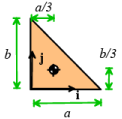M/18 (α2 + b2)

Offer running on EduRev: Apply code STAYHOME200 to get INR 200 off on our premium plan EduRev Infinity!

## Introduction to Dynamics and Vibrations- Notes, Videos, MCQs

20 videos|53 docs

,

,

,

,

,

,

,

,

,

,

,

,

,

,

,

,

,

,

,

,

,

,

,

,

;